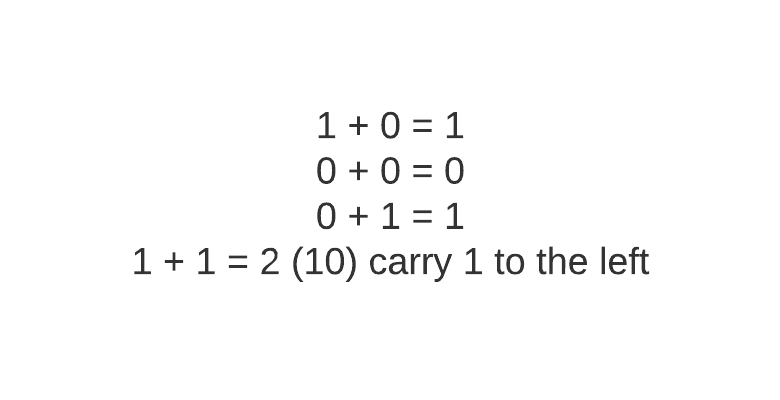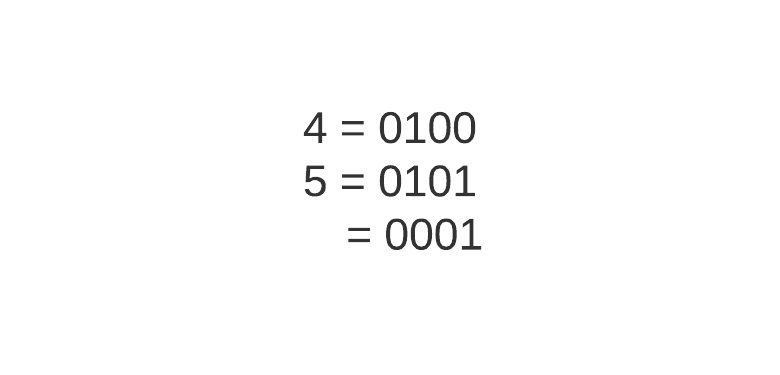XOR is very different from AND and OR. AND and OR is straightforward to understand. I think one of the reasons is probably AND and OR are often used in English words. For instance, when we say AND, it usually meant we want both of the two choices. When we say OR, we typically mean one or the other or both. However, XOR blend AND and OR together to produce what’s called “exclusive or.”

What is exclusive or?

When you want either one or the other, but not both. For instance, if you’re going to choose to buy candy or chocolate, but you don’t want to buy both.

So why is XOR so magical?

Before I talk about the magic that XOR brings, I want you to know that we don’t need XOR for any of our operations. We can just technically represent XOR and AND and OR. I let you ponder upon that one, or you can also search that on Stack Overflow. Therefore, instead of writing long lines of AND’s and ORs to represent XOR, you can just write XOR.

Does that mean XOR is for programmers who want to condense their code to more succinct? In other words, that programmer who writes one-liner without commenting on their code?

If it can condense a long operation into an XOR, it will be great. I saw code based on if statements that they will use XOR to check both entities. When you learn a vocabulary superb, which means super happy, and you use that in your essays. It is succinct, and it is more declarative. However, if a non-English native read your sentence, they might not understand and need to check on those words.

But, that is not the reason why XOR is magical.

To know why XOR is magical, we have to look at how it treats 0’s and 1’s.

Here are some of the things that I discover when looking at the XOR truth table:

If we do 1 XOR 0, it will be equal to 1. If we do 0 XOR 0, it will be equal to 0. If we do 1 XOR 1, it will be equal to 0. If we do 0 XOR 1, it will be equal to 1.

Did you notice any pattern here?

If you don’t, replace the 1’s with x.

If we do x XOR 0, it will be equal to x. If we do 0 XOR 0, it will be equal to 0. If we do x XOR x, it will be equal to 0. If we do 0 XOR x, it will be equal to x.

We see that XOR with 0 equals the same number x. However, if we do XOR the same number, it will equal 0. That means XOR 0 is like an identity feature of XOR, and XOR anything with x will flip the left operand to the opposite.

In other words, XOR also helps you check the difference between the two values. You know if both values are the same if you XOR them. The result is 0. On the other hand, you know if they are different because it won’t be 0.

In terms of 0’s and 1’s, XOR, either 0’s or 1’s will result in that number - identity operation. XOR 0’s or 1’s with 1’s will flip that number.

You see, XOR has more use cases than a simple bit. First, it helps us check if and only if the two operands are different. Second, it helps us identify what difference contains in both values.

These two can help us solved lots of use cases. I’m going to specify three here.

## Finding the Unique Values in an Array

This is a famous one.

Let’s say there are arrays in the array that all elements appear in pairs. You want to check if the collection has a unique element.

Remember, if you XOR element by itself, you get 0 as a result. If you XOR element with some other element with 0, you will get that other element. Therefore, we can XOR all the elements in the array. XOR the same number results in 0, and XOR 0 will result in that element.

## Sum without Carry

This is the one that blows my mind.

I didn’t know that XOR two bits are equal to adding both numbers without the carrying number.

Here is the rules for simple addition between two bits:Do you notice if we replace addition with XOR, it is the same as the truth table above?

Let that sink in.

If we XOR operation with four and five, 0100 and 0101, we will get 0001, 1. Nine in binary representation is 1001. The 1 in the left-most bit is the one that is carried.## In Place Swapping

How do you swap two variables without using a temporary variable?

x = 2 (10), y = 3 (11)
x = x ^ y (10 ^ 11 = 01 = x)
y = x ^ y (01 ^ 11 = 10 = y)
x = x ^ y (01 ^ 10 = 11 = x )


Let me explain.

When we do XOR on both elements, the 0s will not change the left operand’s value, but 1 will flip the left operand’s bit. Therefore, the above operation can be condensed into this:

k = x^y
y = k ^ y = x ^ y ^ y
z = k ^ y
x = x ^ z = x ^ k ^ y = x ^ (x ^ y ^ y) ^ y


The above problem is the same concept as the unique elements now.

That’s it! Let’s conclude with what we’ve talked about.

## Conclusion

XOR is an operator for exclusive or. It means given two boolean values, we want either one or the other, but not both. XOR can be represented by AND’s and ORs. However, if you know XOR by now, why don’t you use it in your codebase? ;)

XOR on 0 serves as an identity operator. If you XOR a with 0, it will return a. If you XOR the same number, it will result in 0. Therefore, we can use this property to find any unique elements in an array, the sum of integers without a carry, and swapping two values in place.

If you have any other XOR trick on your belt, don’t hesitate to comment on the comment section below so everyone can learn from you.

#### Related Posts

##### Explain Cut Vertex and Cut Edges like Im Five

Finding a critical component in a network will not as hard as your think

##### Solving the Muddy City Problem with Minimum Spanning Tree

Mnimum Spanning Tree

##### Everything You want to know about Graph Coloring is Here

Introduction to Graph Coloring

##### Common Pattern of Creating Stack-Safe Recursion

Create your recursion in a stack safe way with Tampolining

##### Data Structure for Spatial Indexing

Spatial Indexing requires a different data structure than a 1D space